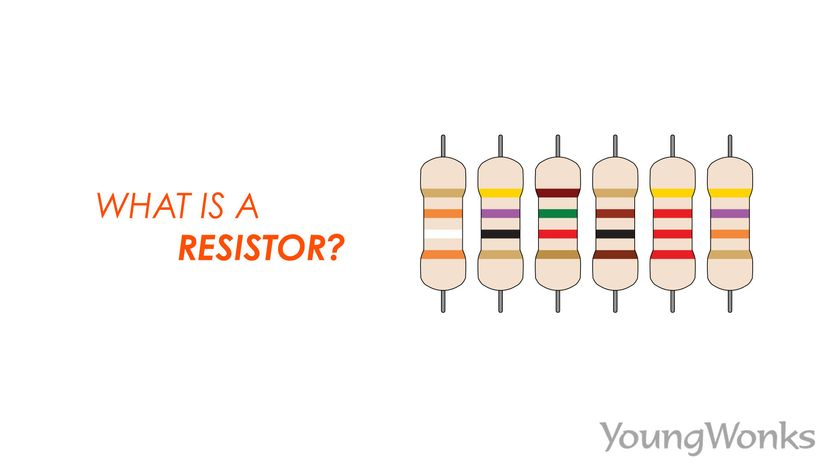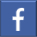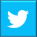# What is a Resistor?

## Where we look at resistors, how they function, their key applications and more…Jan 13, 2022    By Team YoungWonks *

What is a resistor? When we learn about electronics and basic circuits, resistors are, without a doubt, a key component; other electronic components include transistors, inductors, capacitors and diodes. All of these are connected to each other using conductive wires or traces. In this blog, we shall look at resistors, what they do, how they function and their major applications.

## What is a resistor?

A resistor is a passive two-terminal electrical component of a circuit that implements electrical resistance in the circuit; resistance in turn is a measure of the opposition to current flow in an electrical circuit. Thus resistors are basically used to decrease current flow, adjust signal levels, divide voltages, bias active elements, and end transmission lines, among other things.

The resistance implemented by resistors is calculated in ohms (Ω). An ohm is the resistance that occurs when a current of one ampere (A, or I) passes through a resistor with a one volt (V) drop across its terminals. The current is proportional to the voltage across both ends of the terminal. The ratio is represented by Ohm’s law that states that: R = V/ I, where V is the voltage, I is the current and R is the resistance.

Today, resistors are used for many purposes, including limiting electric current, generating heat, dividing voltage, matching and loading electronic circuits and setting time constants. Available in the markets with resistance values over a range of more than nine orders of magnitude, resistors can be tinier than a square millimeter as seen in many electronic items and they could also be used as electric brakes to chip away kinetic energy from trains.

The international IEC (International Electrotechnical Commission, an international standards organization) symbol for a resistor is a rectangular shape with leads at each end as seen below:However, in the US, the fixed resistor is typically symbolized by a zigzag line as seen below:## How does a resistor work?

A resistor’s function is to restrict/ limit the flow of electrical current and it carries out its function in one of three ways: one is through the use of  a less conductive material, another involves making the conductive material thinner and lastly. It works by making the conductive material longer. Many resistors today are wire-wound; here there’s a conductive wire wound around an insulating middle, while other resistors instead have a spiral of carbon, called carbon-film. Wire-wound resistors are typically more precise and stable than carbon-film ones, the resistance of these resistors is controlled by the number of turns and thickness of the wire. And while there are several types of resistors out there, they all operate as per the same three principles shared above.

Let us look at how a resistor works through the use of an example. Check the circuit diagram below:In this example, we have a 15 volt d.c. supply for a 3 volt LED. Without a current limiting resistor or even in case of low resistance (which would mean more current flowing through the circuit), the LED will burn. So we use a resistor that goes on to limit the current and cause a voltage drop. What then should the resistance of the resistor be in order to do so?

So to start with, we see that the circuit has 15 volts power supply, out of which 3 volts are used by the LED, this means that there’s 12 volts for the resistor. We also know that the circuit must have a current of about 20 milliamps (0.02 amps) else the LED will burn out.

We now use the Ohm’s Law to find the resistance (R) needed to protect the LED from excessive current. Ohm’s Law is V = I×R

So substituting the values here, we get

12 = 0.02×R

Therefore, R = 12 ÷0.02

Thus,      R = 600 Ω

Using Ohm’s Law we see that we need a 450 ohm resistor for the LED to not burn.

## Finding the resistance of a resistor

Resistor color code is commonly used to find the resistance of a resistor. The color coding method is quite simple, where each color is associated with a number between 0 and 9.In the resistor color band chart shown above, we see a resistor where the first band is blue, the second and third bands are red and the fourth band is gold. Now, the first color blue is associated with 6 and the second color red is associated with the number 2. Placing these two numbers (6 and 2) next to each other in the corresponding order will give you the two-digit number 62. To this, we add two zeros on the right as the third color red is associated with the number 2. This gives us a resistor value of 6200 ohms. You can also write it as 6.2 kiloohms or 6.2 KΩ.

You can read about the resistor color coding technique in detail in this blog: https://www.youngwonks.com/blog/Finding-the-resistance-of-a-resistor

And you can check out our video about understanding resistor color code here:## Different types of resistors

Resistors come in different shapes and sizes. The most common types are through-hole and surface mount resistors. A resistor can be static, standard resistor, special, or a variable resistor.

The two basic types of resistors are linear resistors and non-linear resistors.

### Linear resistors

Linear resistors are resistors whose values change with changes in applied temperature and voltage. There are two types of linear resistors: fixed resistors and variable resistors.

Fixed resistors have a specific value and these values cannot be changed. Carbon composition resistors, wire wound resistors, thin film resistors and thick film resistors all come under fixed resistors.

Variable resistors, meanwhile, are the resistors that do not have a specific value and the values can be changed with the help of dial, knob, and screw. These resistors find applications in radio receivers for controlling volume and tone. Potentiometers, rheostats and trimmers are all variable resistors.

### Non-linear resistors

Non-linear resistors are the ones whose values change as per the temperature and voltage applied and they are not dependent on Ohm’s law. Thermistors, varistors and photo resistors are different types of non-linear resistors.

## Resistor applications

Resistors have many applications today even as we live in a world increasingly dependent on electrical appliances and devices.

For instance, resistors are used for controlling temperature and voltmeter; they are also used in digital multimeters, amplifiers, modulators, demodulators, transmitters and oscillators.

Photo resistors are used in photographic devices, flame detectors and burglar alarms, whereas wire wound resistors have high power applications are they are used in situations where there’s a need for balanced current control, high sensitivity, and accurate measurement.

## Uses of resistor

### Resistors used in circuit functions

Many types of resistors work as per the usage range. Here one can set the resistance value by using a feature called a knob. Changes in the resistance value affect the flow of current inside the circuit.
Such resistors that are used in circuit functions are typically used to control the speed of a motor, the pitch of a musical tone or even the loudness of an amplifier.

### Resistors used in LEDs and transistors

As seen in the example above, excessive current flowing through LEDs and transistors can pose a serious problem. They are dangerous and LEDs can even burn, which is where resistors come in. Using resistors in such circuits helps ensure that the LEDs and transistors, and other types of semiconductors operate in the current range ideal for them.

### Resistors used for heating

When a light bulb is turned on in a circuit, the metal filament glows white-hot owing to the extremely high temperature generated from the resistance (slow electricity flow rate) when electricity is passed through it. Indeed, a lot of heat is produced when conducting current and this is why resistors are used in many heating appliances such as heaters, toasters, microwaves and electric stoves.

### Resistors used to operate at a particular time and at a specific frequency

Many circuits use a resistor connected to a capacitor in order to access the timing source. Circuits in devices such as light flashers, electronic sirens and blinking lights make use of this feature.

These devices are typically used in airplanes and tall towers as collision avoidance measures. They fill up current till a certain time and then discharge light. The resistor determines how much current has to be allowed through at a given time. So if the resistance in the circuit goes up, the time for discharging current in the circuit also shoots up. The operating principle here is that the capacitor holds an electric charge like a bucket holds water and it takes a specified time to fill with current, while the resistance decides how fast the capacitor is filled.

### Resistors used for diving voltage

There are situations when some components are needed to function in a much lesser voltage than the supplied input voltage. Here dividing the voltage (the potential difference) is a good solution: connecting the resistors in a series helps reduce the voltage across each resistor equally, thereby making the appliance run smoothly even in such conditions.

*Contributors: Written by Vidya Prabhu; Lead image by: Abhishek Aggarwal

This blog is presented to you by YoungWonks. The leading coding program for kids and teens.

YoungWonks offers instructor led one-on-one online classes and in-person classes with 4:1 student teacher ratio.

Sign up for a free trial class by filling out the form below:

By clicking the "Submit" button above, you agree to the privacy policyShare on FacebookShare on Twitter
help